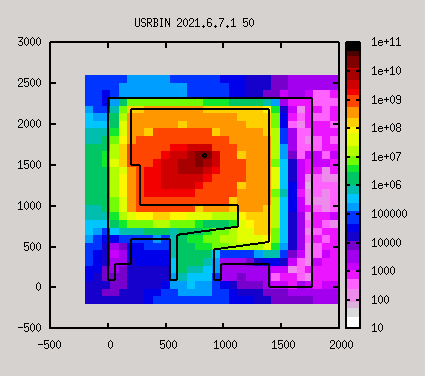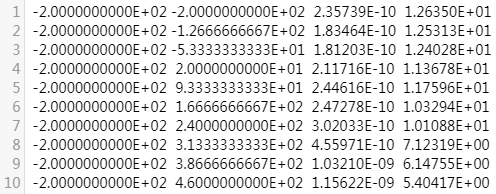# How to change the position of the geometry layer on a USRBIN plot

Dear expert,
The following are the files and plot results of the two simulations I did.

2021.5.28(XZ).flair (3.8 KB)
2021.5.28（XZ）.inp (4.2 KB)
2021.6.7.1.flair (4.6 KB)
2021.6.7.1.inp (3.5 KB)

In the two plot results , one of them could see the geometric outline vaguely, and the other could not see the geometric outline at all.
What is the reason? How do I set Flair if I want to see a clear geometric outline in the image?

Dear Junjie,

the geometry layer is automatically generated at the position calculated as the average of the minimum and maximum projection limits. The projection limits are by default the minimum and maximum of the USRBIN scorings, but they can be manually adjusted.

NOTE: It is important to set the projection limits reasonably, as the presented values are averages between the limits! See slide 53-57 of

If you want to have a geometry layer at a different height, you can specify it’s position with the Pos parameter at the marked section of the plot window:

Cheers,
David

Dear expert,

I set POS to 678 and there are clear lines in the plot result.
Does the current plot result represent the dose equivalent at altitude 678?
Do numerical results represent average results? Does it change as Settings change?Dear Junjie,

setting the Pos parameter doesn’t change the values of the plot, it only sets which height the geometry is plotted at.

The numerical values - in your case - are the averages for the given X-Y coordinates along the Z axis between the limits (-200 cm and 1200 cm).

Cheers,
David

Does setting Norm have no effect on numerical results,too?

If I want to get a dose equivalent at a specified height, how should I set it?

Dear @Junjie_Zhang,
You should really have a look at the slides that @horvathd indicated to you. There you will find the answer to all these type of questions. You can also search for previous questions in the forum, a lot of these question have already been asked and answered.

1 Like